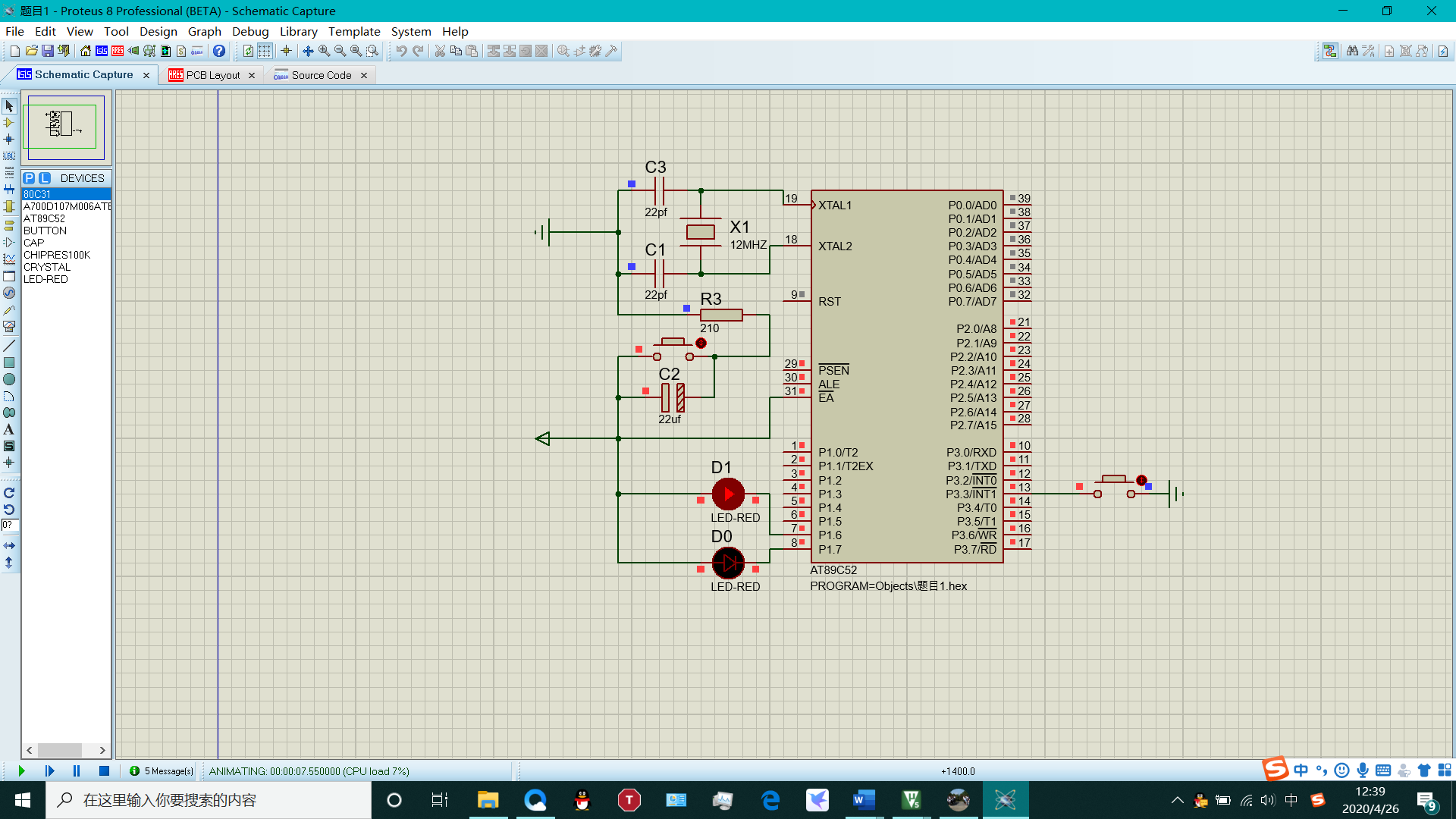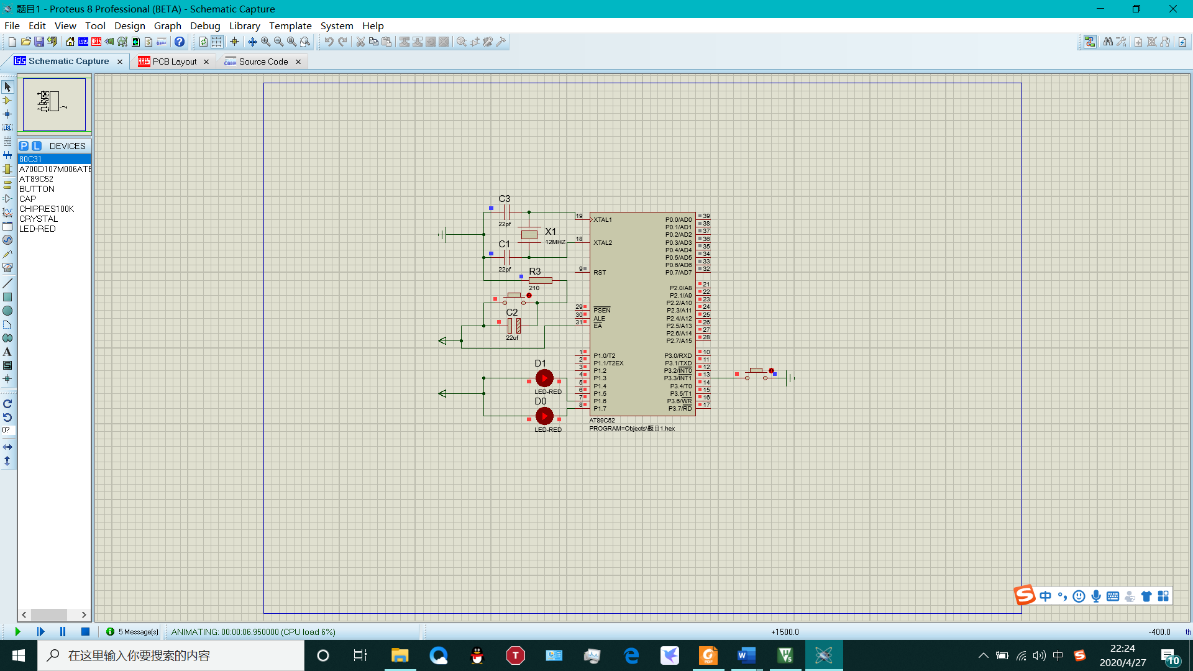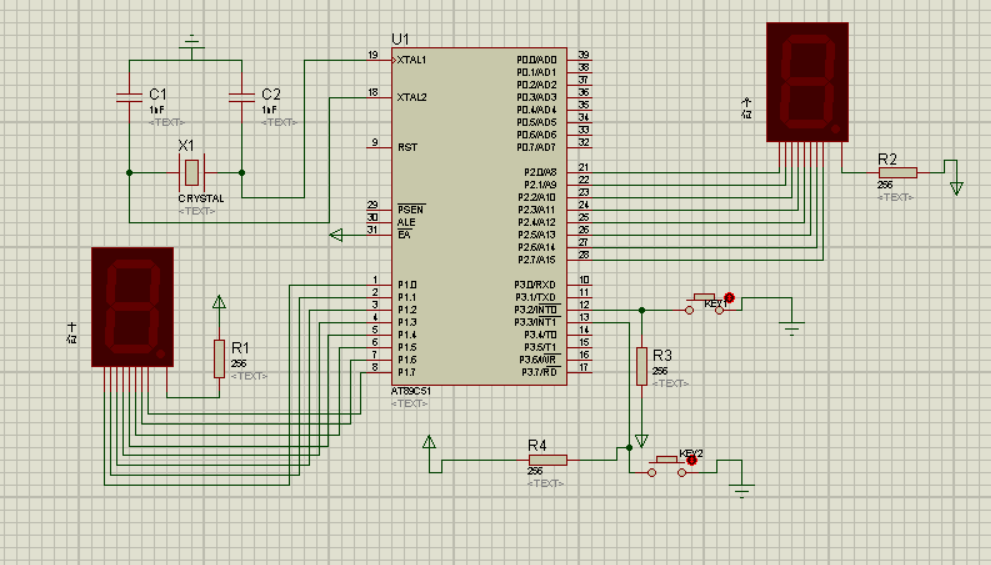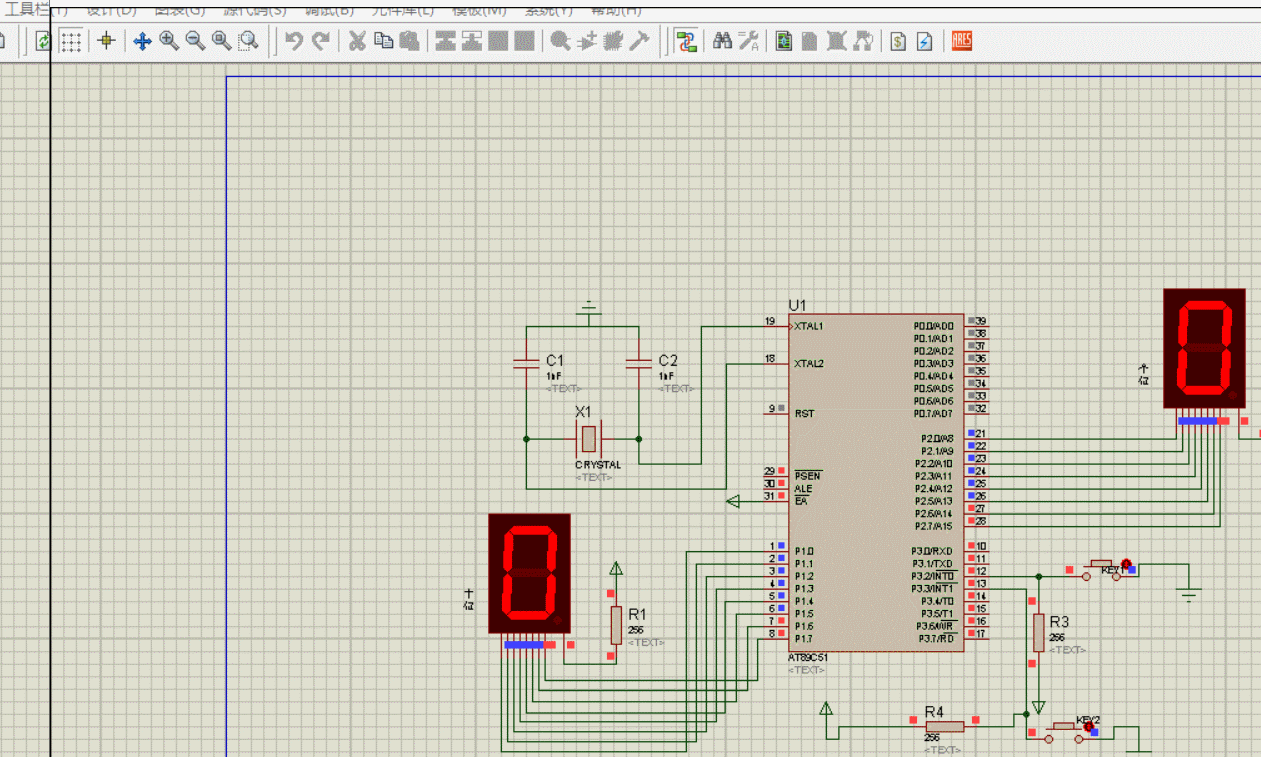• 按下一个键与同时按下两个键的时候，led灯闪烁的效果不一样（文档内有注释）
• 定时器中断控制流水灯延时时间，外部中断0控制按键实现切换流水灯延时时间，外部中断1控制实现切换流水灯方向；
• 设计一个通过触发外部中断INT1实现的在0-3之间计数（四进制）的计数器，并通过P1.6和P1.7端口上的LED，显示计数的值。
1.实验题目：
设计一个通过触发外部中断INT1实现的在0-3之间计数（四进制）的计数器，并通过P1.6和P1.7端口上的LED，显示计数的值。
2.KEIL代码
#include <reg51.h>
sbit P16=P1^6;
sbit P17=P1^7;
void INT1_Init();//声明中断初始化函数
void main()
{
INT1_Init();//调用中断初始化函数
while(1);
}
void INT1_Init() //编写中断初始化函数
{
EA=1;//开启中断总开关
EX1=1;//开启外部中断1
IT1=1;//设定为下降沿触发
IE1=0;//清零中断标志位
}
void INT1_Rupt() interrupt 2//编写外部中断1服务函数
{
static int i=1;
switch (i)
{
case 1:
P16=1;P17=1;i++;break;
case 2:
P16=1;P17=0;i++;break;
case 3:
P16=0;P17=1;i++;break;
case 4:
P16=0;P17=0;i=1;break;
default:;
}
}


3.Protues仿真
LED灯显示效果为10时截图：LED灯显示效果为11时截图：展开全文嵌入式 物联网 keil mdk
• 51单片机：利用外部中断实现按键按一下数码管数字加1直到加到99，另一个按键实现清零 题目要求： 利用外部中断实现按键按一下数码管数字加1，实现00-99的循环，另一个按键实现清零，不管数码管数字是多少，该按键按...
51单片机：利用外部中断实现按键按一下数码管数字加1直到加到99，另一个按键实现清零
题目要求：

利用外部中断实现按键按一下数码管数字加1，实现00-99的循环，另一个按键实现清零，不管数码管数字是多少，该按键按下数码管显示00

准备工作：
keil建立工程proteus仿真验证
仿真电路图：代码如下：
/*
*功能：开关key1控制实现每按一次，数码管数字加1，实现00-99的循环
*开关key2实现随时清零
*/

#include<reg52.h>
sbit SEG1 = P1^0;  //定义显示十位的数码管
sbit SEG2 = P2^0;  //定义显示个位的数码管
sbit key1 = P3^2;  //定义实现数码管加1的开关key1
sbit key2 = P3^3;  //定义实现清零的开关key2
typedef unsigned int uint;
uint i,j;
uint sum = 0;
unsigned char code DSY_CODE[] = {0xC0,0xF9,0xA4,
0xB0,0x99,0x92,
0x82,0xF8,0x80,0x90}; //共阳极数码管
void display(uint i,uint j); //数码管显示函数

void main()
{
IE = 0X85;
IT0 = 1;
IT1 = 1;
while(1)
{
display(i,j);
}

}

void EX0_INT(void) interrupt 0	// 外部中断0，实现按键按一下数码管数字加一
{
sum++;
}

void EX1_INT(void) interrupt 2//外部中断1，实现清零
{
sum = 0;
}

void display(uint i,uint j)	//数码管显示数字
{
i = sum/10;			//数码管显示十位数字
j = sum%10;		    //数码管显示个位数字
if(sum == 100)
sum = 0;
P1 = DSY_CODE[i];
P2 = DSY_CODE[j];
}


仿真结果：展开全文• 单片机外部中断实现外部按键，对于微机c语言也有借鉴意义
• C５１单片机——多个按键中断控制实现 １．实现代码 /* * 2018-10-22 */ #include&lt;STC15F2K60S2.h&gt; #define uchar unsigned char #define uint unsigned int sbit KEY_1 = P3^2; sbit KEY_2 = P3^3; ...
C５１单片机——多个按键中断控制实现
１．实现代码
/*
* 2018-10-22
*/
#include<STC15F2K60S2.h>
#define uchar unsigned char
#define uint unsigned int

sbit KEY_1 = P3^2;
sbit KEY_2 = P3^3;
sbit KEY_3 = P1^7;
sbit led_sel = P2^3;

uchar keysta[] = {1,1,1};//the current state of the key
uchar led[] = {0x80,0x40,0x20,0x10,0x08,0x04,0x02,0x01}; //The light settings
uchar i;  //number of keys
uchar j;  //number of keys
uchar backup = {1,1,1};

void Init()
{
P0M0 = 0xff; //push-pull output
P0M1 = 0x00;
P2M0 = 0xff;
P2M1 = 0x00;

TMOD = 0x01; //mode selection , way 1
EA = 1;      //Open total interrupt
TH0 = 0xf8;  //the high octet setting of the timer
TL0 = 0xcd;  //the low octet setting of the timer
ET0 = 1;     //start timer interruption
TR0 = 1;     //starting switch

led_sel = 1;
P0 = 0;      //initialize
}

void InterruptTimer0() interrupt 1
{
static uchar keybuf = {0xff,0xff,0xff};//key buffer initial value

TH0=0xf8; //The initial value is loaded each time
TL0=0xcd;

//key values into buffer
keybuf = (keybuf << 1)|KEY_1;
keybuf = (keybuf << 1)|KEY_2;
keybuf = (keybuf << 1)|KEY_3;

//update the key
for(i=0;i<3;i++)  //Loop check
{
if(keybuf[i] == 0x00) // move 8 times
{
keysta[i] = 0;
}
else if(keybuf[i] == 0xff)
{
keysta[i] = 1;
}
}
}

void main()
{
Init();

while(1)
{
for(j=0;j<3;j++)  //Loop check
{
if(keysta[j] != backup[j])
{
if(backup[j] == 0)
{
P0 = led[j];
}
}
backup[j]=keysta[j];
}
}
}

２.程序说明 若KEY1被按下，则LED灯L７发光。（其他灯熄灭） 若KEY2被按下，则LED灯L６发光。 若KEY3被按下，则LED灯L５发光。
展开全文• 51单片机按键中断代码，初始化：边沿触发方式下降沿，打开总的中断。防抖动
• 使用中断实现通过编号为8和C的按键控制数码管数字的加减，加至15之后再循环到0，减到0之后保持0不变。 代码有详细的注释： #include sbit WEI=P2^7; sbit DUAN=P2^6; void delay(int t) { while(t--); } ...
本实验的目的：
使用中断实现通过编号为8和C的按键控制数码管数字的加减，加至15之后再循环到0，减到0之后保持0不变。

#include <reg52.h>

sbit WEI=P2^7;
sbit DUAN=P2^6;

void delay(int t)
{
while(t--);
}
unsigned char code dofly_DuanMa={0x3f,0x06,0x5b,0x4f,0x66,0x6d,0x7d,0x07,0x7f,0x6f};// 显示段码值0~9
unsigned char code dofly_WeiMa[]={0xfe,0xfd,0xfb,0xf7,0xef,0xdf,0xbf,0x7f};//分别对应相应的数码管点亮,即位码

unsigned char KeyScan(void)  //键盘扫描函数，使用行列逐级扫描法
{
unsigned char Val;
P3=0xf0;//高四位置高，低四位拉低
if(P3!=0xf0)//表示有按键按下
{
//DelayMs(10);  //去抖
if(P3!=0xf0)
{           //表示有按键按下
P3=0xfe; //检测第一行
if(P3!=0xfe)
{
Val=P3&0xf0;
Val+=0x0e;
while(P3!=0xfe);

while(P3!=0xfe);
return Val;
}
P3=0xfd; //检测第二行
if(P3!=0xfd)
{
Val=P3&0xf0;
Val+=0x0d;
while(P3!=0xfd);

while(P3!=0xfd);
return Val;
}
P3=0xfb; //检测第三行
if(P3!=0xfb)
{
Val=P3&0xf0;
Val+=0x0b;
while(P3!=0xfb);

while(P3!=0xfb);
return Val;
}
P3=0xf7; //检测第四行
if(P3!=0xf7)
{
Val=P3&0xf0;
Val+=0x07;
while(P3!=0xf7);

while(P3!=0xf7);
return Val;
}
}
}
return 0xff;
}

/*------------------------------------------------
按键值处理函数，返回扫键值
------------------------------------------------*/
unsigned char KeyPro(void)
{
switch(KeyScan())
{
case 0xee:return 0;break;//0 按下相应的键显示相对应的码值
case 0xde:return 1;break;//1
case 0xbe:return 2;break;//2
case 0x7e:return 3;break;//3
case 0xed:return 4;break;//4
case 0xdd:return 5;break;//5
case 0xbd:return 6;break;//6
case 0x7d:return 7;break;//7
case 0xeb:return 8;break;//8
case 0xdb:return 9;break;//9
case 0xbb:return 10;break;//a
case 0x7b:return 11;break;//b
case 0xe7:return 12;break;//c
case 0xd7:return 13;break;//d
case 0xb7:return 14;break;//e
case 0x77:return 15;break;//f
default:return 0xff;break;
}
}

//第一个参数i代表控制第几个数码管，n代表要显示的数字
void Screen1(int i,int n)
{
int high;
P0=0;
DUAN=1;
DUAN=0;

high=n/10;

WEI=1;
P0=dofly_WeiMa[i];
WEI=0;

DUAN=1;
P0=dofly_DuanMa[high];

DUAN=0;

}

void Screen2(int i,int n)
{
int lower;
P0=0;
DUAN=1;
DUAN=0;

lower=n%10;
WEI=1;
P0=dofly_WeiMa[i];

WEI=0;

DUAN=1;
P0=dofly_DuanMa[lower];

DUAN=0;

}

int num;
int Key_push;
int flag;
int main()
{
num=0;
WEI=0;
DUAN=0;

EA=1;
EX0=1;
IT0=0;

EX1=1;
IT1=0;

flag=0;
while(1)
{
if(flag && num>9)
{
Screen1(6,num);
flag=0;
}
else
{
Screen2(7,num);
flag=1;
}
Key_push=KeyPro();
}

return 0;
}

//外部中断处理
void inter_sub() interrupt 0 using 1
{

if(Key_push==8)
{
if(num>0)
num--;
}
Key_push=0;

}

void inter_add() interrupt 2 using 1
{

if(Key_push==12)
{
num++;
if(num==16)
num=0;

}
Key_push=0;
}




展开全文c语言 数码管
• 在实际工程常用到中断的算法来实现按键消抖。 思路：启动一个定时中断，每2ms进一次中断扫描按键状态并储存。连续扫描8次后，观察这8次按键状态是否一致。如果一致，即按键没有发生动作，处于稳定状态。 被监测到...
• 名称：计数器中断实现 100 以内的按键计数 说明：本例 T0 计数器中断实现按键技术，由于计数寄存器初值为 1，因此 P3.4 引脚的每次负跳变都会触发 T0 中断实现计数值累加。计数器的清零外部中断 0 控制。
• 其中画红色方框部分为重要函数实现功能 EXIT.c #include "EXTI/EXTI.h" u8 keyvalue = 0; //键值 /** * 功能：初始化外部中断0/1 * 参数：None * 返回值：None */ void initEXTI_0_1(void) { EXTI_...
• 一、使用proteus绘制简单的电路图，用于后续仿真 二、编写程序 /******************************************************************************************************************** ---- @Project: ...
• 在完成交通灯基本功能基础上，当有急救车到达时，两向交通信号为全红，以便让急救车通过。假定急救车通过路口时间为10秒，急救车通过后，交通灯恢复中断前状态。本实验题以按键中断申请，表示有急救车通过。
• 51单片机程序 实现时钟及按键中断的功能，通过protues仿真没问题的TM4C123G
• 在51单片机上通过C语言编写代码，使用中断按键按一下数码管上面的数字从00开始加1，实现00——99的循环 准备工作： keil建立工程 proteus仿真验证 代码如下： /* *功能：用中断实现开关控制实现每按一次，...
• PIC单片机按键中断程序的设计技巧，内含按键 长按键代码及注释！
• 实验大致上就是说：按键不按的时候，灯一直亮，按键按下的时候，灯不亮，报警器响。现在给了汇编语言，要改成c语言，并在proteus里面仿真。 思考与总结： 响应中断请求的条件： 1.总中断允许开关EA=1。 2.中断源的...
• 单片机MSP430G2553的按键非中断控制LED亮灭状态切换程序，其他程序比如非按键中断、定时器中断、PWM等单片机MSP430G2553系列代码我会陆续上传，敬请期待。
• 单片机按键编程，有时会遇到一个问题，多个按键来控制多个死循环，而程序一旦进入循环就跳不出来，此时会想到用中断，而普通单片机一般只有2个外部中断，stc系列的查了一下最多也只有5个外部中断，那要是...
• 以Key4为例，使用定时中断2ms进行消抖，对连续8次（16ms）的按键状态进行判断. 如果全部为1则弹起，将按键当前状态（Keysta）为1； 全部为0则按下，将按键当前状态（Keysta）置0； 其余状态都为抖动，按键当前...Keil
• //判断减一执行后是否开启加一按键 void delay(uint z); void display(); uchar code table[]={0xc0,0xf9,0xa4,0xb0,0x99,0x92,0x82,0xf8,0x80,0x90}; uchar code wei[]={0x01,0x02}; uchar m[]={0,0}; void delay...c语言
• 51单片机：利用两个按键通过中断分别实现数码管上数字自加自减功能（00——99） 功能描述： 开关key1控制实现每按一次，数码管数字加1，实现00-99的循环 开关key2按键按一下实现数码管减1，实现00-99的循环 数码管......